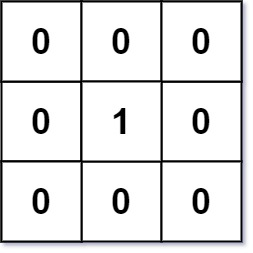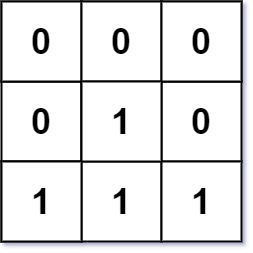0542. 01 矩阵

# 0542. 01 矩阵#

• 标签：深度优先搜索、广度优先搜索
• 难度：中等

## 题目大意 #

• 两个相邻元素间的距离为 $1$。
• $m == mat.length$。
• $n == mat[i].length$。
• $1 \le m, n \le 10^4$。
• $1 \le m * n \le 10^4$。
• $mat[i][j] === 0$ 或者 $mat[i][j] == 1$。
• $mat$ 中至少有一个 $0$。1 2  输入：mat = [[0,0,0],[0,1,0],[0,0,0]] 输出：[[0,0,0],[0,1,0],[0,0,0]]1 2  输入：mat = [[0,0,0],[0,1,0],[1,1,1]] 输出：[[0,0,0],[0,1,0],[1,2,1]] 

## 解题思路 #

### 思路 1：广度优先搜索 #

1. 使用一个集合变量 visited 存储所有值为 0 的元素坐标。使用队列变量 queue 存储所有值为 0 的元素坐标。使用二维数组 res 存储对应位置元素（即 $mat[i][j]$）到最近的 $0$ 的距离。
2. 我们从所有为如果队列 queue 不为空，则从队列中依次取出值为 0 的元素坐标，遍历其上、下、左、右位置。
3. 如果相邻区域未被访问过（说明遇到了值为 1 的元素），则更新相邻位置的距离值，并把相邻位置坐标加入队列 queue 和访问集合 visited 中。
4. 继续执行 2 ~ 3 步，直到队列为空时，返回 res

### 思路 1：代码 #

  1 2 3 4 5 6 7 8 9 10 11 12 13 14 15 16 17 18 19 20 21 22 23 24 25 26  import collections class Solution: def updateMatrix(self, mat: List[List[int]]) -> List[List[int]]: rows, cols = len(mat), len(mat) res = [[0 for _ in range(cols)] for _ in range(rows)] visited = set() for i in range(rows): for j in range(cols): if mat[i][j] == 0: visited.add((i, j)) directions = {(1, 0), (-1, 0), (0, 1), (0, -1)} queue = collections.deque(visited) while queue: i, j = queue.popleft() for direction in directions: new_i = i + direction new_j = j + direction if 0 <= new_i < rows and 0 <= new_j < cols and (new_i, new_j) not in visited: res[new_i][new_j] = res[i][j] + 1 queue.append((new_i, new_j)) visited.add((new_i, new_j)) return res 

### 思路 1：复杂度分析 #

• 时间复杂度：$O(m \times n)$。
• 空间复杂度：$O(m \times n)$。